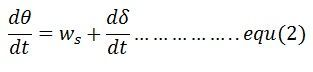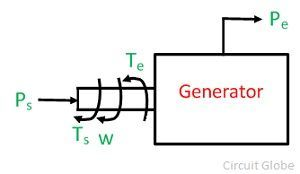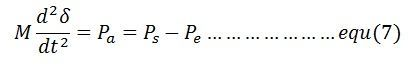# Swing Equation

The transient stability of the system can be determined by the help of the swing equation. Let θ be the angular position of the rotor at any instant t. θ  is continuously changing with time, and it is convenient to measure it with respect to the reference axis shown in the figure below. The angular position of the rotor is given by the equationθ – angle between rotor field and a reference axis
ws – synchronous speed
δ – angular displacement

Differentiation of equation (1) givesDifferentiation of equation (2) givesPower flow in the synchronous generator is shown in the diagram below. If the damping is neglected the accelerating torques, Ta in a synchronous generator is equal to the difference of mechanical input shaft and the electromagnetic output torque, i.e.,Ta – accelerating torque
Ts – shaft torque
Te – electromagnetic torque

Angular momentum of the rotor is expressed by the equation

w- the synchronous speed of the rotor
J – moment of inertia of the rotor
M – angular momentum of the rotor

Multiplying both the sides of equation (5) by w we get

Ps – mechanical power input
Pe – electrical power output
Pa – accelerating power

But,Equation (7) gives the relation between the accelerating power and angular acceleration. It is called the swing equation. Swing equation describes the rotor dynamics of the synchronous machines and it helps in stabilizing the system.